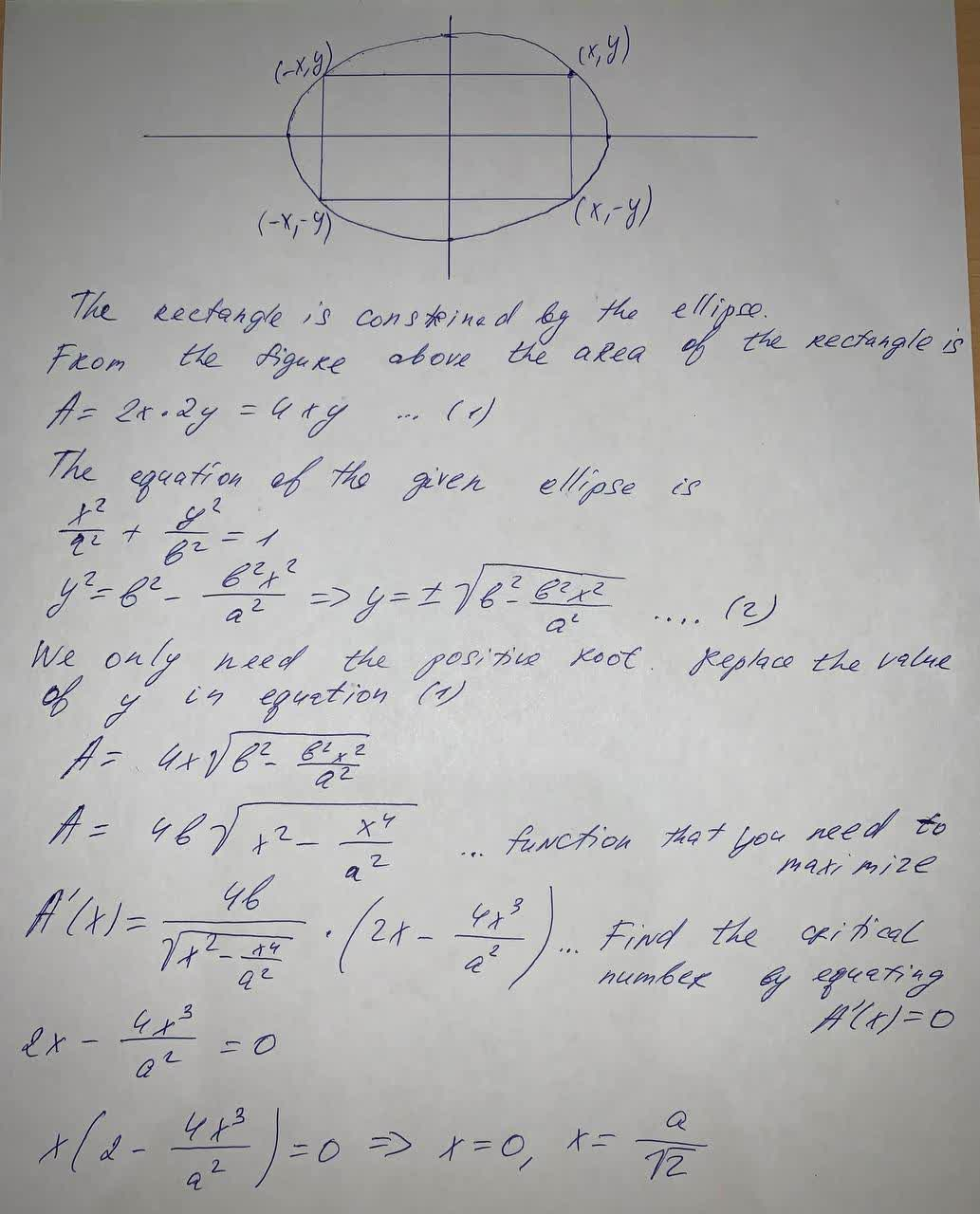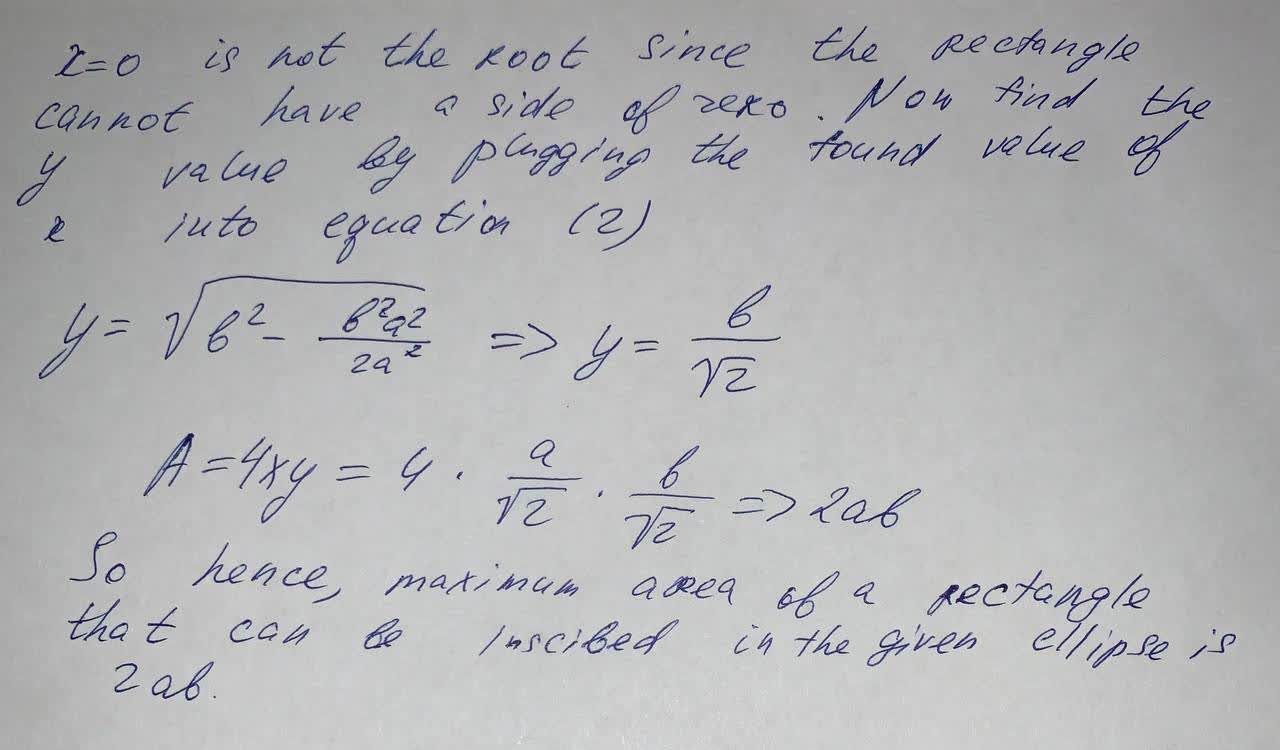find the area of the largest rectangle that can be inscribed in the ellipse \frac{x^2}{a^2}+\frac{y^2}{b^2}=1Chardonnay Felix 2021-06-08 Answered
find the area of the largest rectangle that can be inscribed in the ellipse $$\frac{x^2}{a^2}+\frac{y^2}{b^2}=1$$

• Questions are typically answered in as fast as 30 minutes

Solve your problem for the price of one coffee

• Math expert for every subject
• Pay only if we can solve itTheodore Schwartz
See the photo below: